# 事情是这样的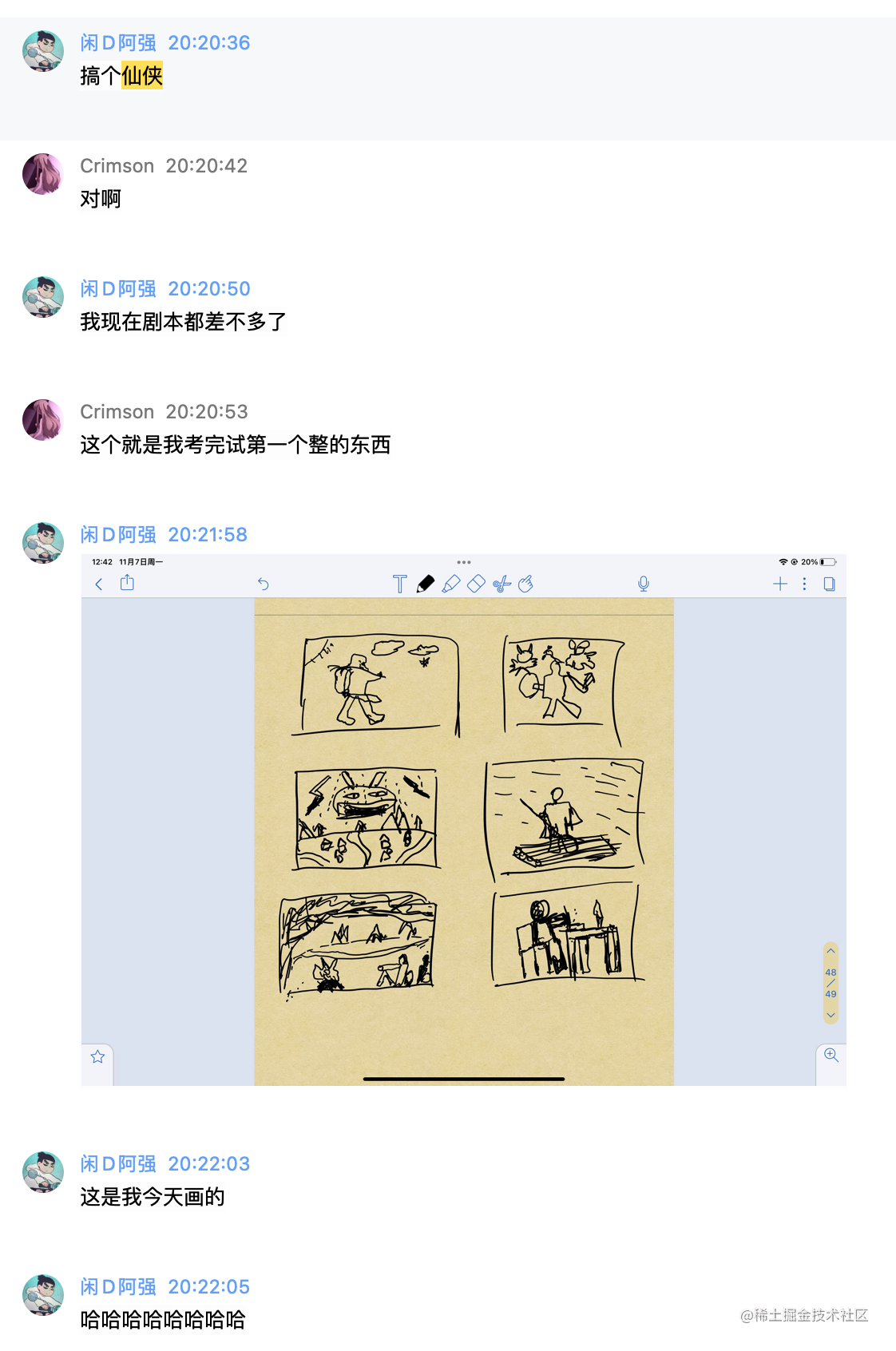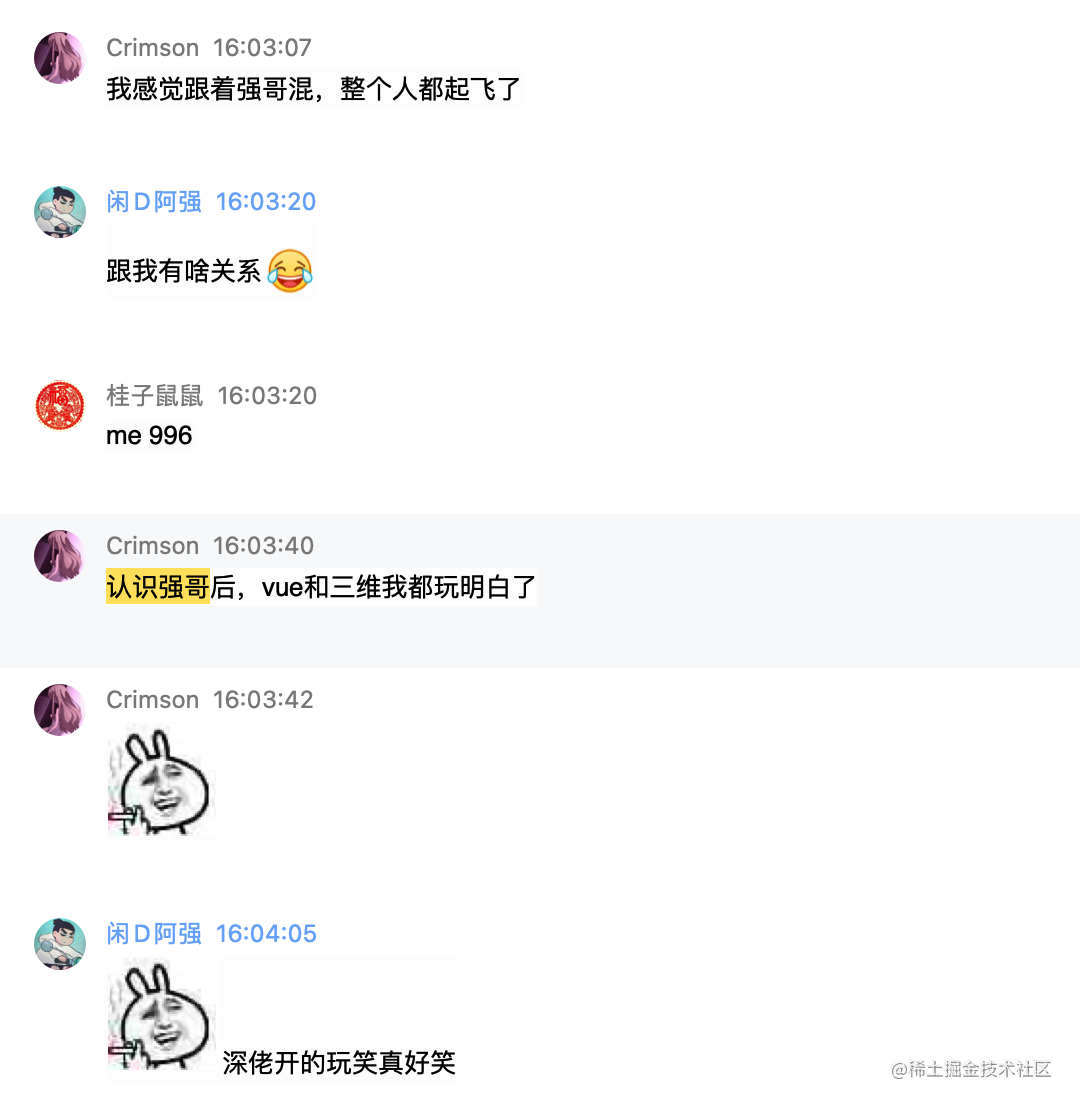# 速通babylon基础功能

## 创建场景

### 创建并获得canvas节点

html ```html

`**JS**`js let canvas = document.getElementById("renderCanvas"); ```

### 创建一个engine引擎

`有了canvas就能创建engine了`

### 可以创建场景了

`有了engine，就能创建场景了`

## 创建一个盒模型，天空和光

`有了场景，就能在里面加入模型，天空和光了`

### 创建盒模型

```js const box = BABYLON.MeshBuilder.CreateBox("box", {}, scene);``` 效果图：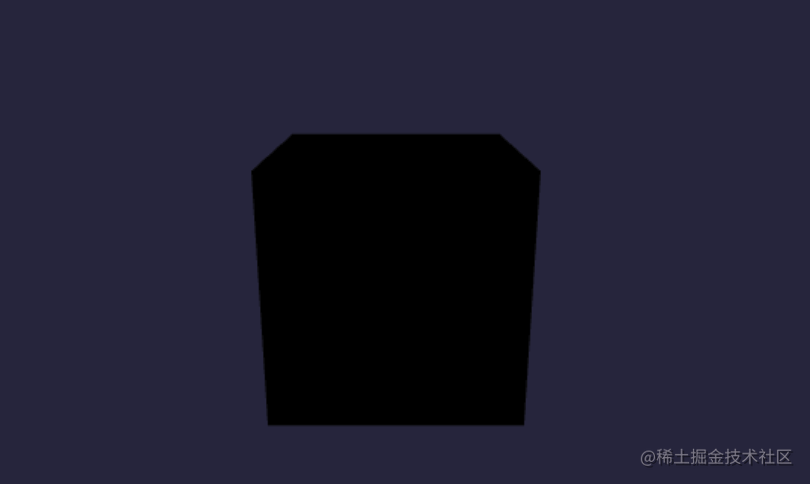### 创建蓝天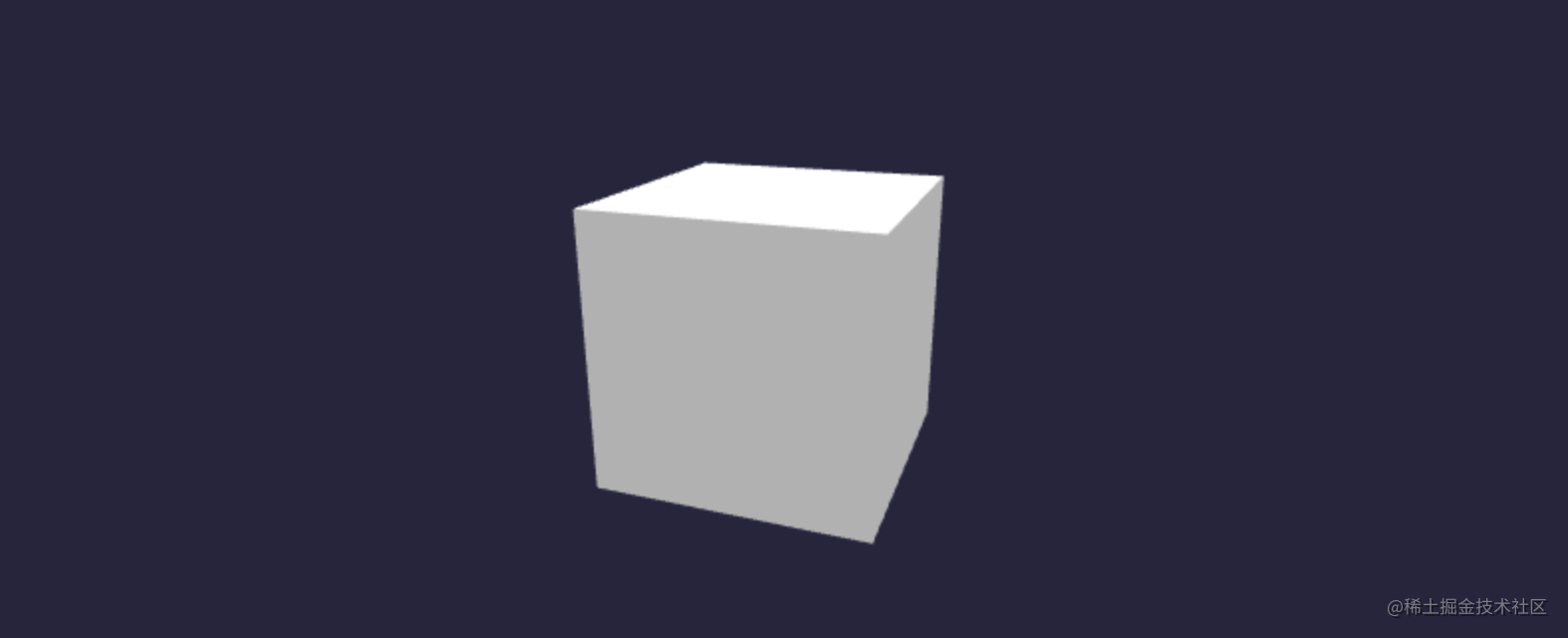• 使用`BABYLON.MeshBuilder.CreateBox`创建，
• 第一个参数是模型的名字
• 第二个是参数，其中仅仅设置可size为1000，代表着，width（宽），hight（高），depth（深）同时设置了1000
• inclination表示太阳的日照，区间为[-0.5,0.5],
• backFaceCulling这个叫剔除，你不设置false，看不到天空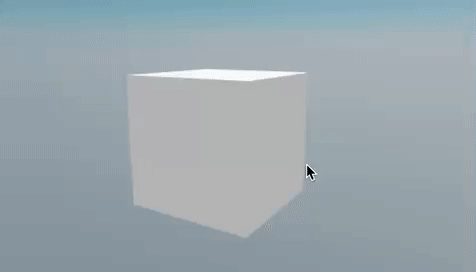### 给盒模型添加材质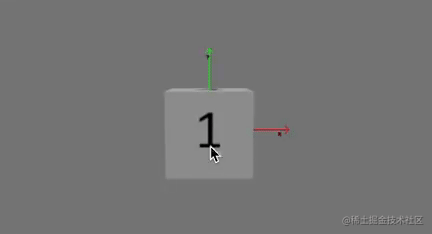faceUV就是配置六个面显示的数组

```js let faceUV = [ new Vector4(1 / 4, 0, 2 / 4, 1), // 0号面 new Vector4(1 / 4, 0, 2 / 4, 1), // 1号面 new Vector4(2 / 4, 0, 3 / 4, 1), // 2号面 new Vector4(2 / 4, 0, 3 / 4, 1), // 3号面 new Vector4(0, 0, 1 / 4, 1), // 4号面 new Vector4(3 / 4, 0, 1, 1), // 5号面 ];```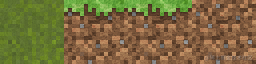em～～～，我其实也搞不懂，这个四维坐标是咋回事，但我将亲测出来的理解写下来，希望对你有用： - x和z，横向来看，你可以理解x表示截取图片的开始，z表示结束 - y和w，纵向来看，你可以理解y是为截取图片的开始，w表示结束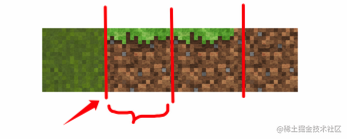• 纵向从0也就是图片的最下方。到1，也就是最高点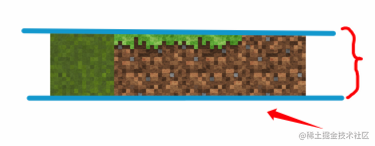# 游戏设计

## 架构设计

### 架构图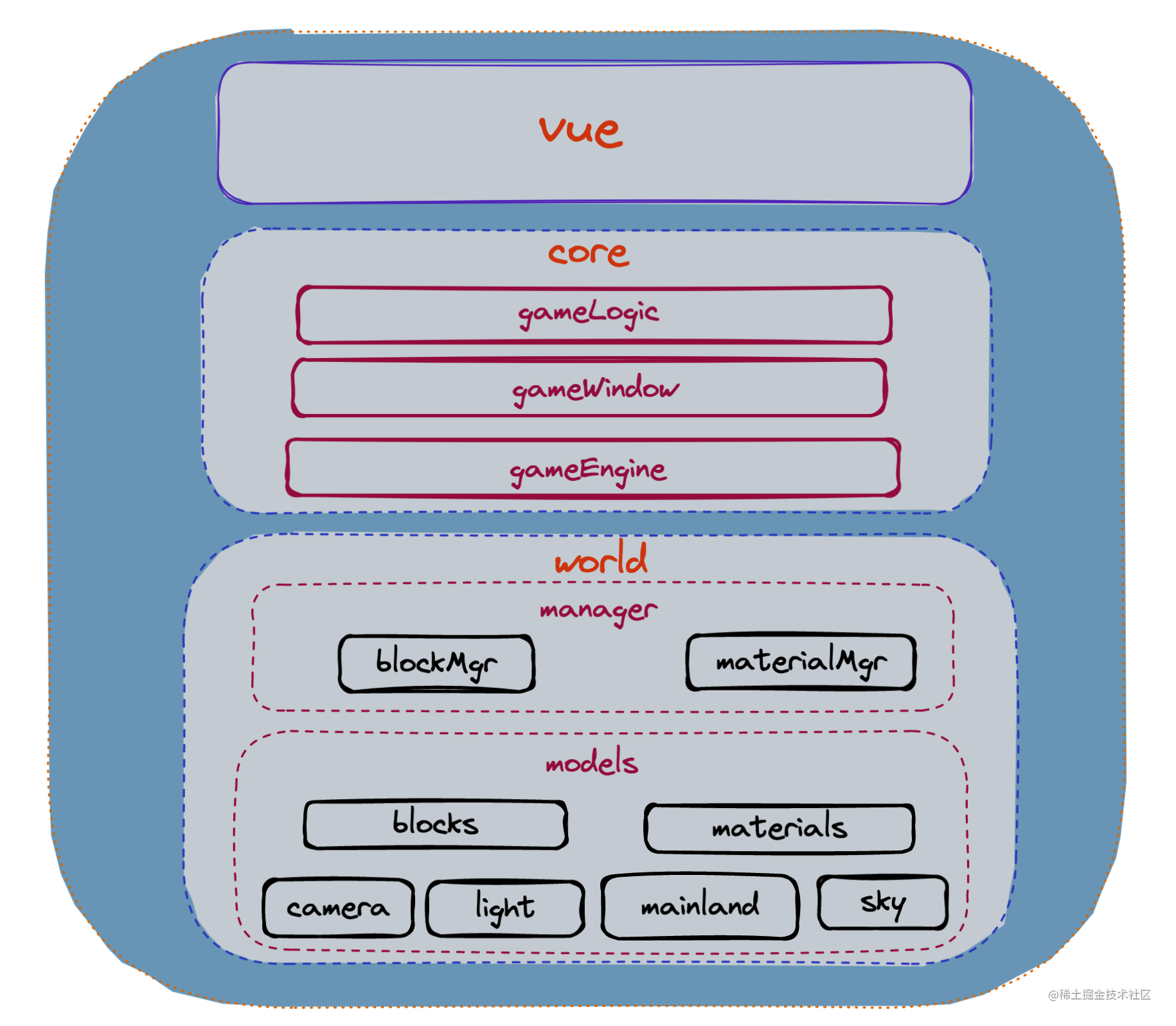### 职能划分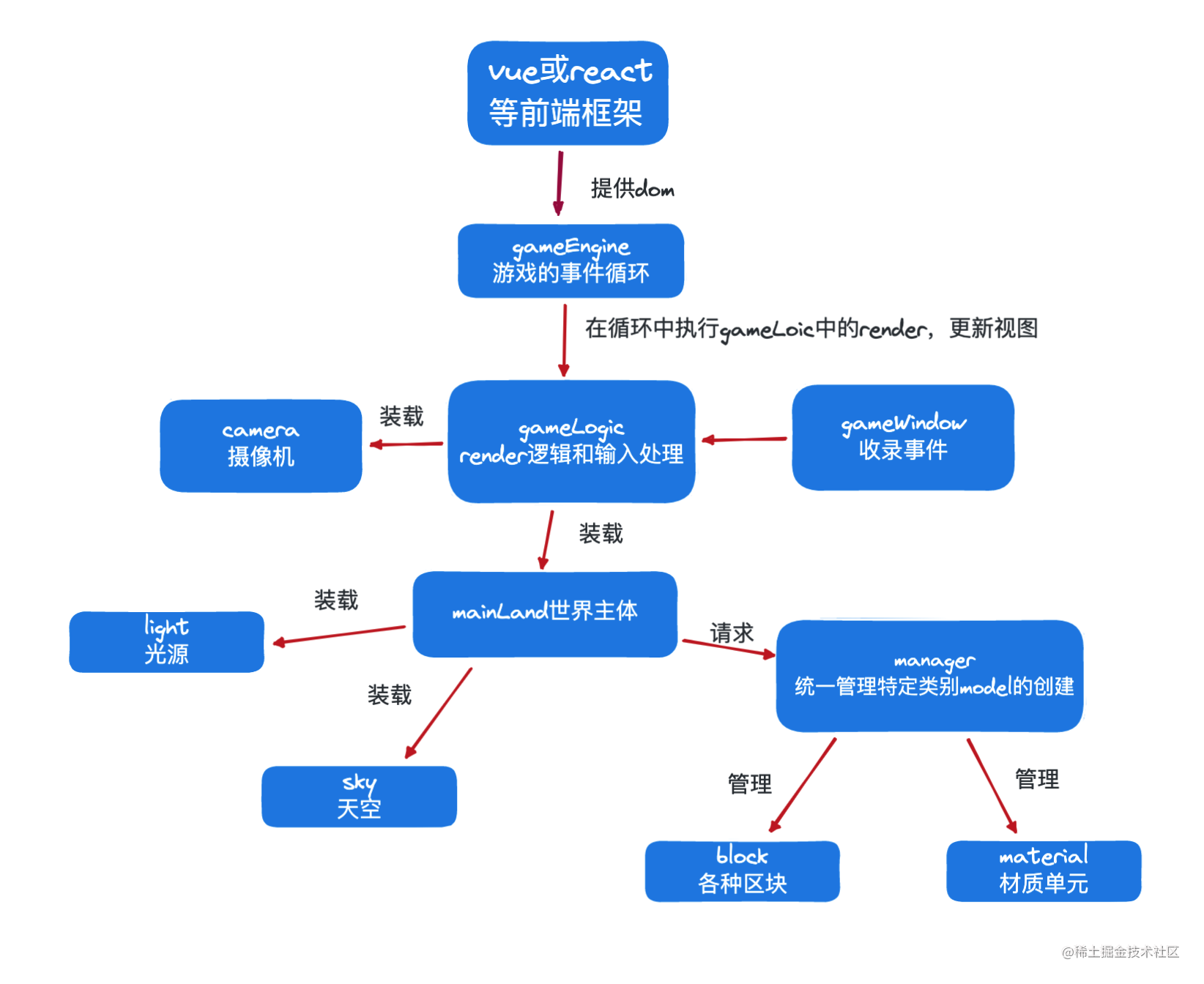### 项目结构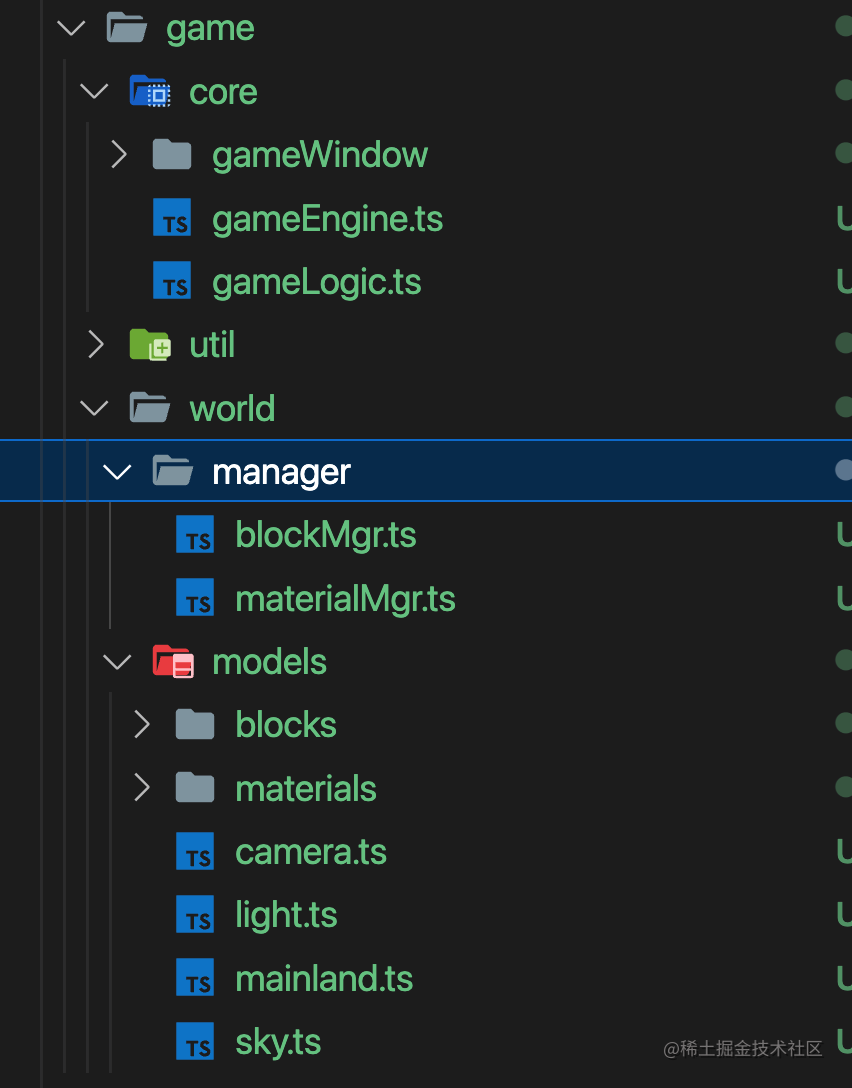## 功能设计

### 创建地图，通过噪声算法

`因为，我们需要一个办法，一个可以生成一个随机种子，往后通过这个种子就能生成固定的数据，并且具备连续性，数据是平滑的。`

``````private seed: number;

// 实例化一个随机数生成器，seed=随机数种子，默认当前时间
constructor(seed?: number) {
this.seed = (seed || Date.now()) % 999999999;
}

// 返回0~1之间的数a
next() {
this.seed = (this.seed * 9301 + 49297) % 233280;
return this.seed / 233280.0;
}

// 返回0~max之间的数
nextInt(max: number) {
return Math.floor(this.next() * max);
}
``````

}

export class PerlinNoiseGenerator {

``````private static readonly F2: number = 0.3660254037844386;
private static readonly G2: number = 0.21132486540518713;
private static readonly F3: number = 1.0 / 3.0;
private static readonly G3: number = 1.0 / 6.0;
[1, 1, 0], [-1, 1, 0], [1, -1, 0], [-1, -1, 0],
[1, 0, 1], [-1, 0, 1], [1, 0, -1], [-1, 0, -1],
[0, 1, 1], [0, -1, 1], [0, 1, -1], [0, -1, -1],
[1, 0, -1], [-1, 0, -1], [0, -1, 1], [0, 1, 1]
];
private readonly PERM: Array<number> = [];

constructor(
seed: number,
option: { octaves: number, persistence: number, lacunarity: number }
) {
this.octaves = option.octaves;
this.persistence = option.persistence;
this.lacunarity = option.lacunarity;
let random = new Random(seed);
for (let i = 0; i < 512; i++) {
this.PERM.push(random.nextInt(256));
}
}

private dot3(v1: Array<number>, v2: Array<number>): number {
return v1 * v2 + v1 * v2 + v1 * v2;
}

private noise2(x: number, y: number): number {
let i1, j1, I, J;
let s = (x + y) * PerlinNoiseGenerator.F2;
let i = Math.floor(x + s);
let j = Math.floor(y + s);
let t = (i + j) * PerlinNoiseGenerator.G2;
let xx = [];
let yy = [];
let f = [];
let noise = [0.0, 0.0, 0.0];
let g = [];
xx = x - (i - t);
yy = y - (j - t);
i1 = xx > yy ? 1 : 0;
j1 = xx <= yy ? 1 : 0;
xx = xx + PerlinNoiseGenerator.G2 * 2.0 - 1.0;
yy = yy + PerlinNoiseGenerator.G2 * 2.0 - 1.0;
xx = xx - i1 + PerlinNoiseGenerator.G2;
yy = yy - j1 + PerlinNoiseGenerator.G2;
I = i & 255;
J = j & 255;
g = this.PERM[I + this.PERM[J]] % 12;
g = this.PERM[I + i1 + this.PERM[J + j1]] % 12;
g = this.PERM[I + 1 + this.PERM[J + 1]] % 12;
for (let c = 0; c <= 2; c++) {
f[c] = 0.5 - xx[c] * xx[c] - yy[c] * yy[c];
}
for (let c = 0; c <= 2; c++) {
if (f[c] > 0) {
noise[c] = f[c] * f[c] * f[c] * f[c] *
}
}
return (noise + noise + noise) * 70.0;
}

private noise3(x: number, y: number, z: number): number {
let c, o1, o2, g = [], I, J, K;
let f = [], noise = [0.0, 0.0, 0.0, 0.0];
let s = (x + y + z) * PerlinNoiseGenerator.F3;
let i = Math.floor(x + s);
let j = Math.floor(y + s);
let k = Math.floor(z + s);
let t = (i + j + k) * PerlinNoiseGenerator.G3;
let pos: Array<Array<number>> = [[], [], [], []];
pos = x - (i - t);
pos = y - (j - t);
pos = z - (k - t);
if (pos >= pos) {
if (pos >= pos) {
o1 = [1, 0, 0];
o2 = [1, 1, 0];
} else if (pos >= pos) {
o1 = [1, 0, 0];
o2 = [1, 0, 1];
} else {
o1 = [0, 0, 1];
o2 = [1, 0, 1];
}
} else {
if (pos < pos) {
o1 = [0, 0, 1];
o2 = [0, 1, 1];
} else if (pos < pos) {
o1 = [0, 1, 0];
o2 = [0, 1, 1];
} else {
o1 = [0, 1, 0];
o2 = [1, 1, 0];
}
}
for (c = 0; c <= 2; c++) {
pos[c] = pos[c] - 1.0 + 3.0 * PerlinNoiseGenerator.G3;
pos[c] = pos[c] - o2[c] + 2.0 * PerlinNoiseGenerator.G3;
pos[c] = pos[c] - o1[c] + PerlinNoiseGenerator.G3;
}
I = i & 255;
J = j & 255;
K = k & 255;
g = this.PERM[I + this.PERM[J + this.PERM[K]]] % 12;
g = this.PERM[I + o1 + this.PERM[J + o1 + this.PERM[o1 + K]]] % 12;
g = this.PERM[I + o2 + this.PERM[J + o2 + this.PERM[o2 + K]]] % 12;
g = this.PERM[I + 1 + this.PERM[J + 1 + this.PERM[K + 1]]] % 12;
for (c = 0; c <= 3; c++) {
f[c] = 0.6 - pos[c] * pos[c] - pos[c] * pos[c] -
pos[c] * pos[c];
}
for (c = 0; c <= 3; c++) {
if (f[c] > 0) {
noise[c] = f[c] * f[c] * f[c] * f[c] * this.dot3(pos[c], PerlinNoiseGenerator.GRAD3[g[c]]);
}
}
return (noise + noise + noise + noise) * 32.0;
}

public simplex2(x: number, y: number): number {
let freq = 1.0;
let amp = 1.0;
let max = 1.0;
let total = this.noise2(x, y);
for (let i = 1; i < this.octaves; i++) {
freq *= this.lacunarity;
amp *= this.persistence;
max += amp;
total += this.noise2(x * freq, y * freq) * amp;
}
return (1 + total / max) / 2;
}

public simplex3(x: number, y: number, z: number): number {
let freq = 1.0;
let amp = 1.0;
let max = 1.0;
let total = this.noise3(x, y, z);
for (let i = 1; i < this.octaves; ++i) {
freq *= this.lacunarity;
amp *= this.persistence;
max += amp;
total += this.noise3(x * freq, y * freq, z * freq) * amp;
}
return (1 + total / max) / 2;
}
``````

}

```

```

### 只渲染视野范围内的

`既然创建地图的功能已经好了，我们就来制定渲染地图的规则`

• 首先我们肯定不能全部渲染出来，我们需要渲染一部分，那么是哪一部分呢？

• 全部渲染出来，卡爆了
• 肯定是以摄像机这个第一视角为中心，以一个合适的视野距离画圆这个区域最为合理。

• 这个视野距离就会作为半径画圆，目前设置的是36，很流程，扫出来的区域也够大
• 也就是根据摄像机的中心坐标，开始计算一个圆形区域的坐标集合

• 这块就是简单的遍历一个圆内的坐标集合，间隔单位就是grassBlock的宽度默认为1
• 但是画圆有点难度，不如我们用一个取巧的方式，画一个方形，然后写个判定是否在圆内的工具函数来进行筛选，岂不是更容易。

• 先画出一个方形，然后在用算法过滤，就这么简单

### 镜头可以碰撞，移动，跳跃